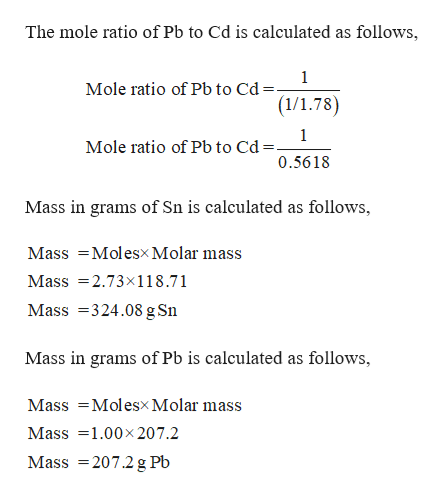# An alloy of Sn, Pb, and Cd has a mole ratio of Sn to Pb of 2.73:1.00, and the mass ratio of Pb to Cd is 1.78:1.00. What is the mass percent of Sn in the alloy?

Question
2 views

An alloy of Sn, Pb, and Cd has a mole ratio of Sn to Pb of 2.73:1.00, and the mass ratio of Pb to Cd is 1.78:1.00. What is the mass percent of Sn in the alloy?

check_circle

Step 1

Given,

An alloy of Sn, Pb, and Cd has a mole ratio of Sn to Pb of ...help_outlineImage TranscriptioncloseThe mole ratio of Pb to Cd is calculated as follows, 1 Mole ratio of Pb to Cd =- (1/1.78) 1 Mole ratio of Pb to Cd . 0.5618 Mass in grams of Sn is calculated as follows, Mass Molesx Molar mass Mass 2.73x118.71 Mass 324.08 g Sn Mass in grams of Pb is calculated as follows, Mass Molesx Molar mass Mass1.00x 207.2 Mass 207.2g Pb fullscreen

### Want to see the full answer?

See Solution

#### Want to see this answer and more?

Solutions are written by subject experts who are available 24/7. Questions are typically answered within 1 hour.*

See Solution
*Response times may vary by subject and question.
Tagged in

### General Chemistry